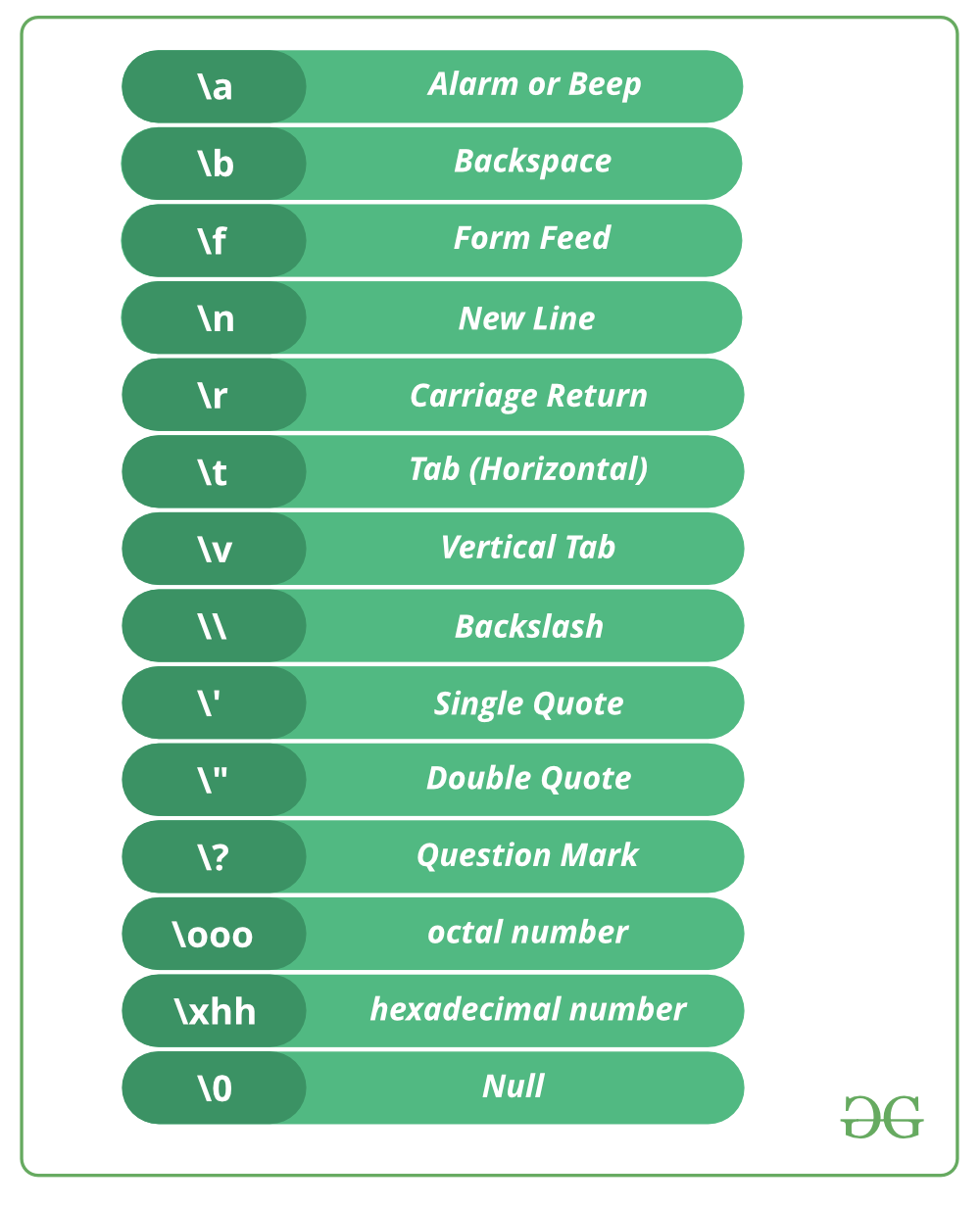Related Articles

# Escape Sequences in C

• Difficulty Level : Easy
• Last Updated : 29 Nov, 2019

In C programming language, there are 256 numbers of characters in character set. The entire character set is divided into 2 parts i.e. the ASCII characters set and the extended ASCII characters set. But apart from that, some other characters are also there which are not the part of any characters set, known as ESCAPE characters.

List of Escape SequencesSome coding examples of escape characters

 `// C program to illustrate``// \a escape sequence``#include ``int` `main(``void``)``{``    ``printf``(``"My mobile number "``          ``"is 7\a8\a7\a3\a9\a2\a3\a4\a0\a8\a"``);``    ``return` `(0);``}`

Output:

```My mobile number is 7873923408.
```
 `// C program to illustrate``// \b escape sequence``#include ``int` `main(``void``)``{``    ``// \b - backspace character transfers``    ``// the cursor one character back with ``    ``// or without deleting on different ``    ``// compilers.``    ``printf``(``"Hello Geeks\b\b\b\bF"``);``    ``return` `(0);``}`

Output:

```The output is dependent upon compiler.
```
 `// C program to illustrate``// \n escape sequence``#include ``int` `main(``void``)``{``    ``// Here we are using \n, which``    ``// is a new line character.``    ``printf``(``"Hello\n"``);``    ``printf``(``"GeeksforGeeks"``);``    ``return` `(0);``}`

Output:

```Hello
GeeksforGeeks```
 `// C program to illustrate``// \t escape sequence``#include ``int``main(``void``)``{``    ``// Here we are using \t, which is``    ``// a horizontal tab character.``    ``// It will provide a tab space ``    ``// between two words.``    ``printf``(``"Hello \t GFG"``);``    ``return` `(0);``}`

Output:

```Hello   GFG
```

The escape sequence “\t” is very frequently used in loop based pattern printing programs.

 `// C program to illustrate``// \v escape sequence``#include ``int` `main(``void``)``{``    ``// Here we are using \v, which``    ``// is vertical tab character.``    ``printf``(``"Hello friends"``);`` ` `    ``printf``(``"\v Welcome to GFG"``);`` ` `    ``return` `(0);``}`

Output:

```Hello Friends
Welcome to GFG
```
 `// C program to illustrate \r escape ``// sequence``#include ``int` `main(``void``)``{``    ``// Here we are using \r, which``    ``// is carriage return character.``    ``printf``(``"Hello fri \r ends"``);``    ``return` `(0);``}`

Output: (Depend upon compiler)

```ends
```
 `// C program to illustrate \\(Backslash)``// escape sequence to print backslash.``#include ``int` `main(``void``)``{``    ``// Here we are using \,``    ``// It contains two escape sequence ``    ``// means \ and \n.``    ``printf``(``"Hello\\GFG"``);``    ``return` `(0);``}`

Output: (Depend upon compiler)

```Hello\GFG
```

Explanation : It contains two escape sequence means it after printing the \ the compiler read the next \ as as new line character i.e. \n, which print the GFG in the next line

 `// C program to illustrate \' escape``// sequence/ and \" escape sequence to``// print single quote and double quote.``#include ``int` `main(``void``)``{``    ``printf``(``"\' Hello Geeks\n"``);``    ``printf``(``"\" Hello Geeks"``);``    ``return` `0;``}`

Output:

```' Hello Geeks
" Hello Geeks
```
 `// C program to illustrate``// \? escape sequence``#include ``int` `main(``void``)``{``    ``// Here we are using \?,  which is ``    ``// used for the presentation of trigraph``    ``// in the early of C programming. But``    ``// now we don't have any use of it.``    ``printf``(``"\?\?!\n"``);``    ``return` `0;``}`

Output:

```??!
```
 `// C program to illustrate \OOO escape sequence``#include ``int` `main(``void``)``{``    ``// we are using \OOO escape sequence, here ``    ``// each O in "OOO" is one to three octal ``    ``// digits(0....7).``    ``char``* s = ``"A\0725"``;``    ``printf``(``"%s"``, s);``    ``return` `0;``}`

Output:

```A:5
```

Explanation : Here 000 is one to three octal digits(0….7) means there must be atleast one octal digit after \ and maximum three.Here 072 is the octal notation, first it is converted to decimal notation that is the ASCII value of char ‘:’. At the place of \072 there is : and the output is A:5.

 `// C program to illustrate \XHH escape ``// sequence``#include ``int` `main(``void``)``{``    ``// We are using \xhh escape sequence.``    ``// Here hh is one or more hexadecimal``    ``// digits(0....9, a...f, A...F).``    ``char``* s = ``"B\x4a"``;``    ``printf``(``"%s"``, s);``    ``return` `0;``}`

Output:

```BJ
```

Explanation : Here hh is one or more hexadecimal digits(0….9, a…f, A…F).There can be more than one hexadecimal number after \x. Here, ‘\x4a’ is a hexadecimal number and it is a single char. Firstly it will get converted into decimal notation and it is the ASCII value of char ‘J’. Therefore at the place of \x4a, we can write J. So the output is BJ.

This article is contributed by Bishal Kumar Dubey. If you like GeeksforGeeks and would like to contribute, you can also write an article using contribute.geeksforgeeks.org or mail your article to contribute@geeksforgeeks.org. See your article appearing on the GeeksforGeeks main page and help other Geeks.